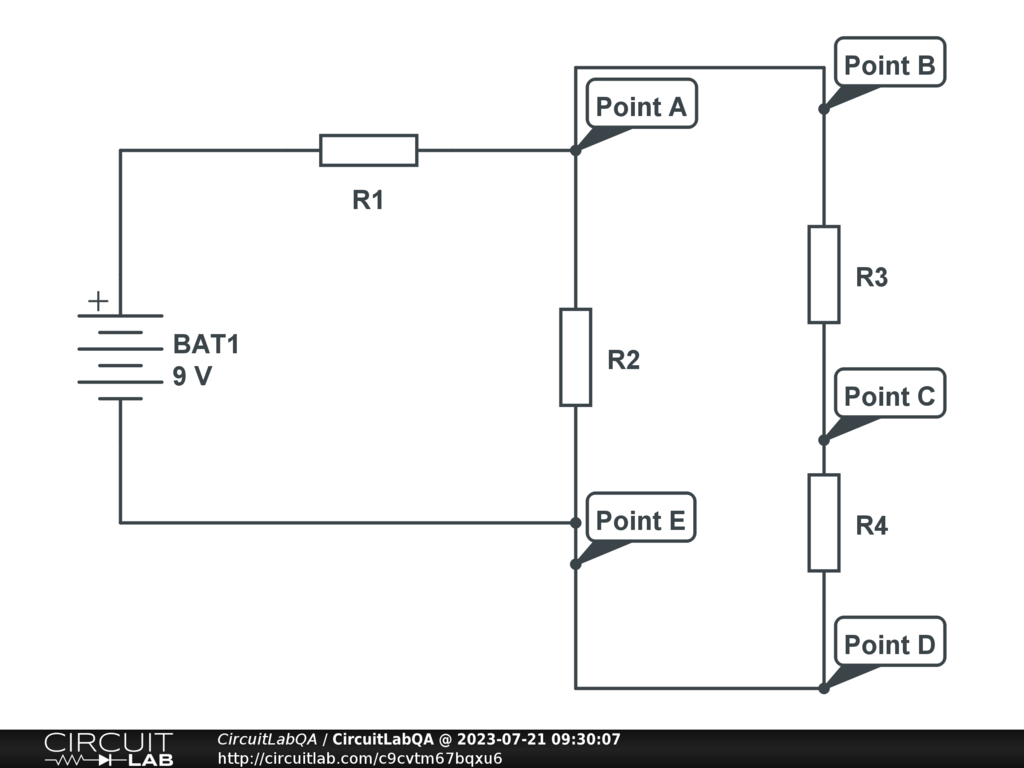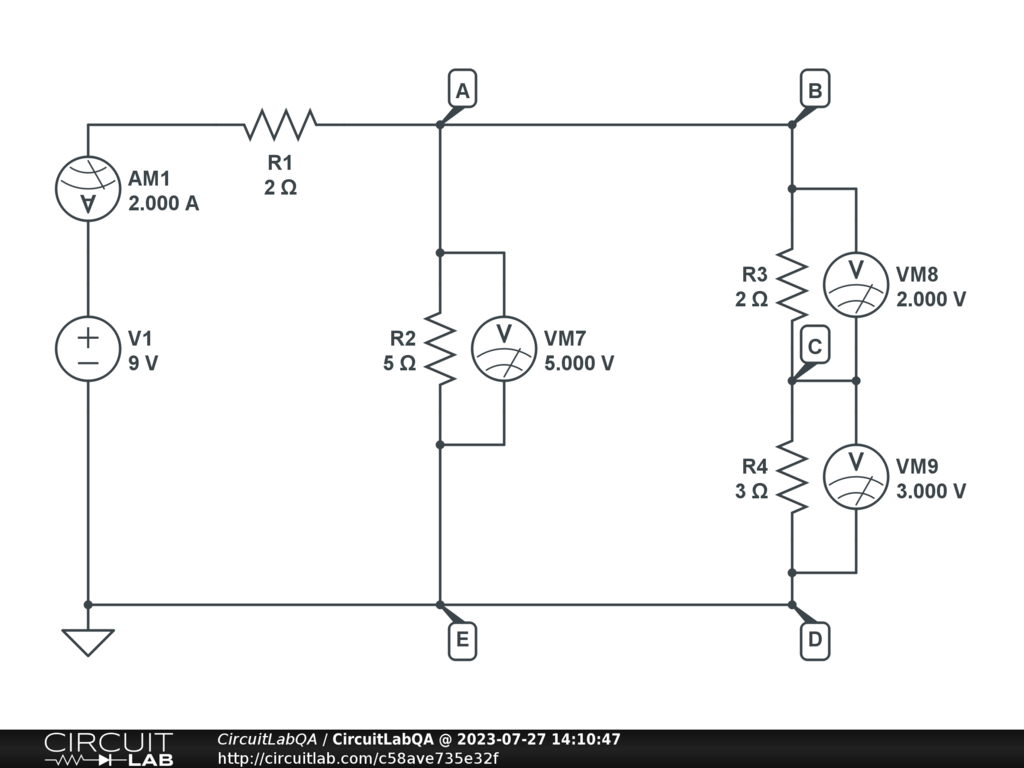## Stuck on question about resistanceHi all, i am relatively new to electronics, i have always had an interest but never studied it, now at 46 I am taking the leap!!! I am reading a book and have come to the end of a specific chapter on resistance, there are questions at the end that I have managed to answer but the last 2 have really stumped me, and i was hoping someone here could help. The first question is: Take a look at the following diagram, if the voltage drop between Point B and C is 2v and the voltage drop between C and D is 3v, what is the Voltage drop between A and E? What is the voltage at Point A and Point E 2nd question: If that circuit runs with 2A total current what is the value of the resistor R1 This has had me perplexed for days now, Any help would be greatly received. by Ericobi July 21, 2023 Add comment... Please sign in or create an account to comment.To answer your first question, when two resistors are in series like R3 and R4 they share the same current, and when resistors are in parallel like R2 and R3&4 they share the same voltage. With that in mind, since the voltage across both R3 and R4 adds up to 5V and R2 is in parallel, R2 shares the same 5V. To answer your second question, you know that the total voltage drop across all R2, R3, & R4 is 5V and the supply is 9V, so the voltage across R1 is 9V - 5V = 4V. Ohms law states that V = I*R and therefore R = V/I = 4V/2A = 2Ohms. The remaining resistances can be found by the current being split evenly between R2 and R3&4 and using the known voltages with ohms law. +1 vote by jbowler12345 July 27, 2023 Add comment... Please sign in or create an account to comment.

 Great that you're learning electronics! Let me see if I can help with these circuit questions: For the first one, we know: drift hunters - Voltage drop BC = 2V - Voltage drop CD = 3V Since those drops are in series, the total voltage drop from A to E is their sum: Voltage drop AE = Voltage BC + Voltage CD = 2V + 3V = 5V We don't know the actual voltage at points A or E, but we know the difference between them is 5V. +1 vote by weberralph September 05, 2023 Add comment... Please sign in or create an account to comment.

CircuitLab's Q&A site is a FREE questions and answers forum for electronics and electrical engineering students, hobbyists, and professionals.

We encourage you to use our built-in schematic & simulation software to add more detail to your questions and answers.

Acceptable Questions:

• Concept or theory questions
• Practical engineering questions
• “Homework” questions
• Software/hardware intersection
• Best practices
• Design choices & component selection
• Troubleshooting

Unacceptable Questions:

• Non-English language content
• Non-question discussion
• Non-electronics questions
• Vendor-specific topics
• Pure software questions
• CircuitLab software support

Please respect that there are both seasoned experts and total newbies here: please be nice, be constructive, and be specific!